# 教程 | 没有博士学位，照样玩转TensorFlow深度学习

1、概述①神经网络的定义及如何训练神经网络

②如何使用 TensorFlow 构建基本的 1 层神经网络

③如何添加多层神经网络

④训练提示和技巧：过拟合、dropout、学习速率衰减等...

⑤如何解决深度神经网络的问题

⑥如何构建卷积网络

①Python 2 或 3（建议使用 Python 3）

②TensorFlow

③Matplotlib（Python 的可视化库）

2. 准备：安装 TensorFlow，获取示例代码

\$ git clone https://github.com/martin-gorner/tensorflow-mnist-tutorial

\$ python3 mnist_1.0_softmax.py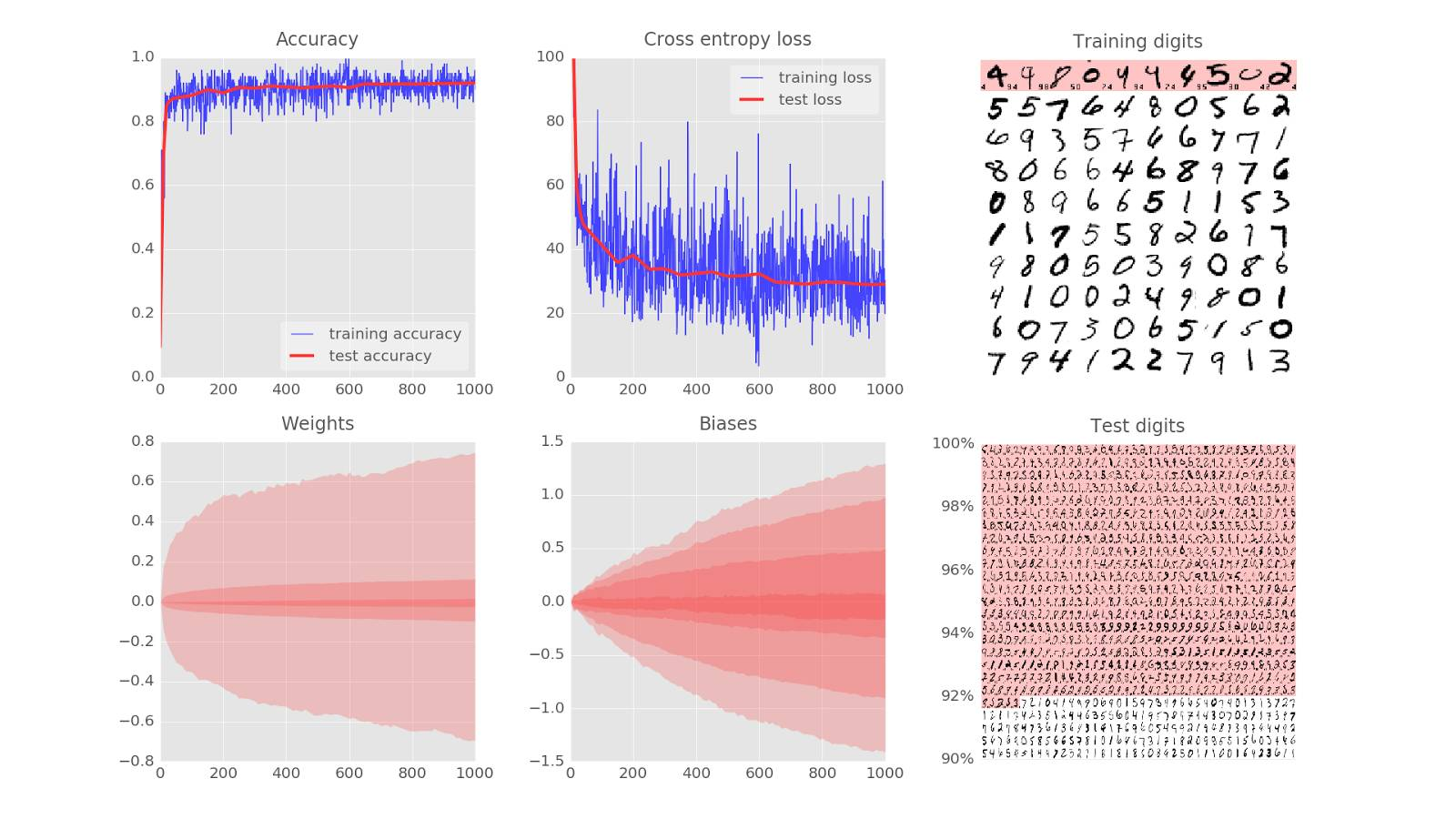3、理论：训练一个神经网络

Training digits => updates to weights and biases => better recognition (loop)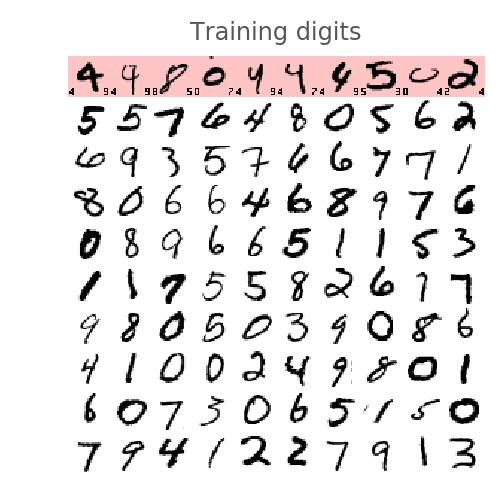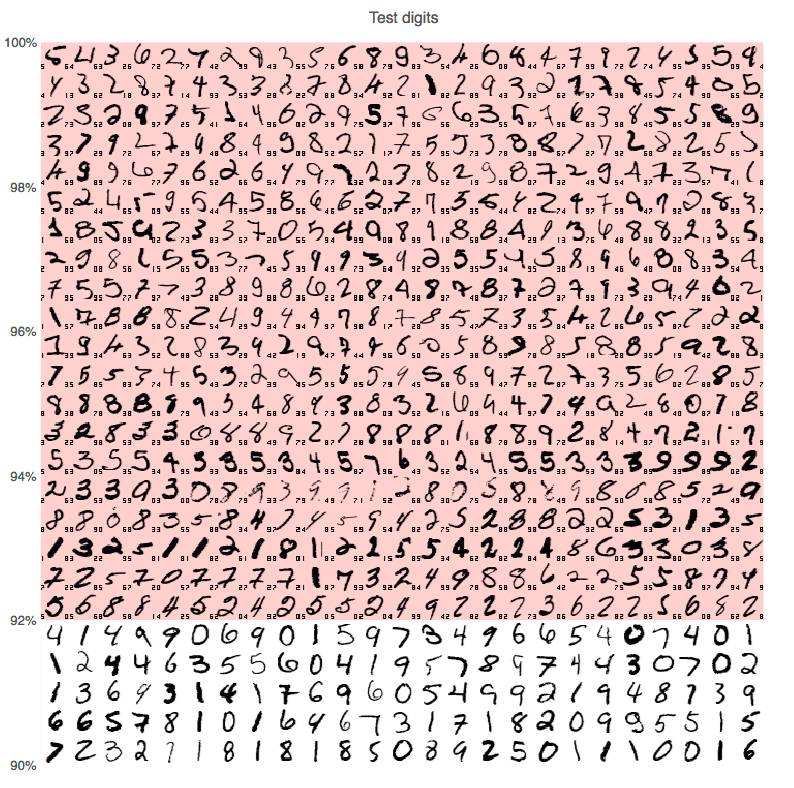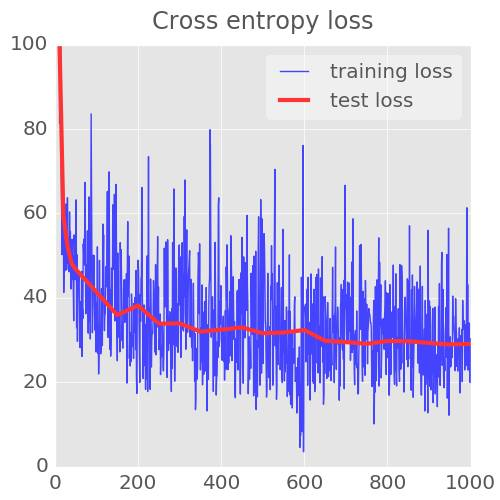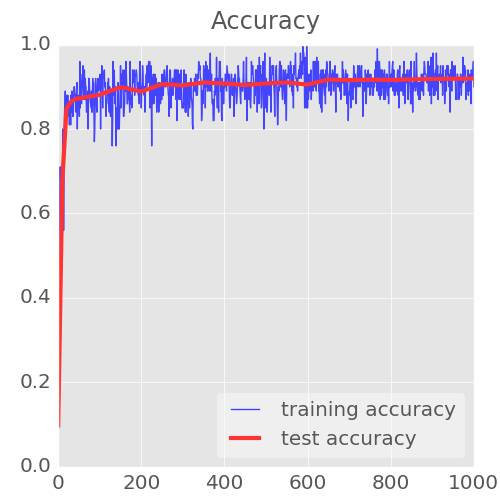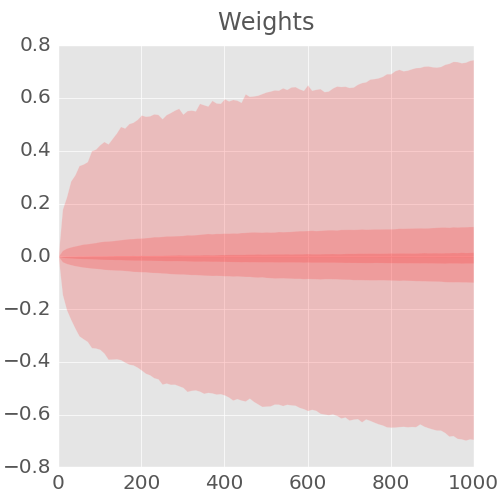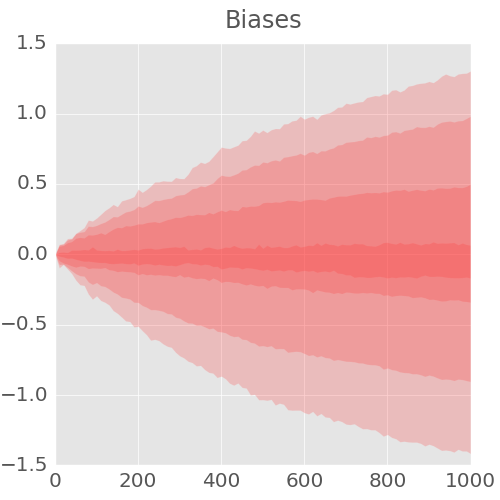1 ......... display 1st graph only 仅显示第 1 张图

2 ......... display 2nd graph only 仅显示第 2 张图

3 ......... display 3rd graph only 仅显示第 3 张图

4 ......... display 4th graph only 仅显示第 4 张图

5 ......... display 5th graph only 仅显示第 5 张图

6 ......... display 6th graph only 仅显示第 6 张图

7 ......... display graphs 1 and 2 显示 1 和 2 图

8 ......... display graphs 4 and 5 显示 4 和 5 图

9 ......... display graphs 3 and 6 显示 3 和 6 图

ESC or 0 .. back to displaying all graphs 返回，显示所有图

O ......... box zoom mode (then use mouse) 框缩放模式（然后使用鼠标）

H ......... reset all zooms 重置所有缩放

Ctrl-S .... save current image 保存当前图像

4、理论 : 单层神经网络MNIST 数据集中，手写数字是 28x28 像素的灰度图像。将它们进行分类的最简单的方法就是使用 28x28=784 个像素作为单层神经网络的输入。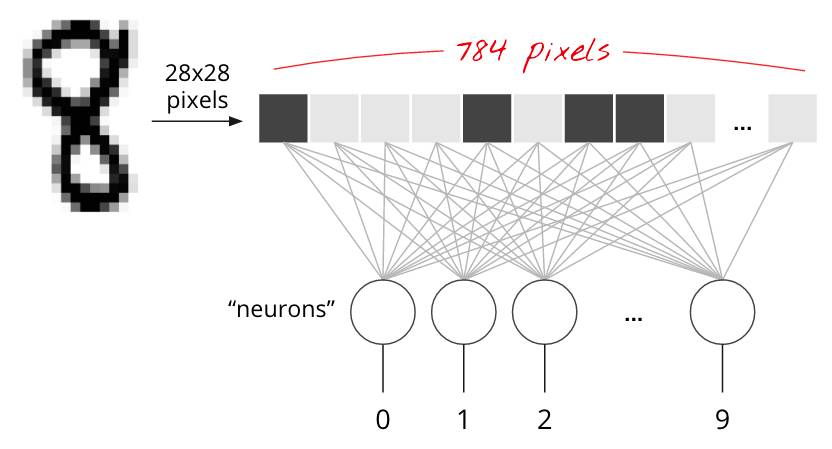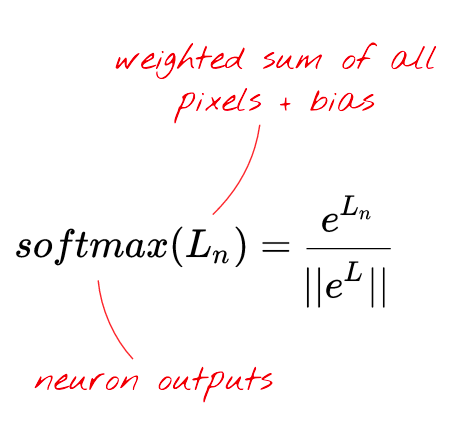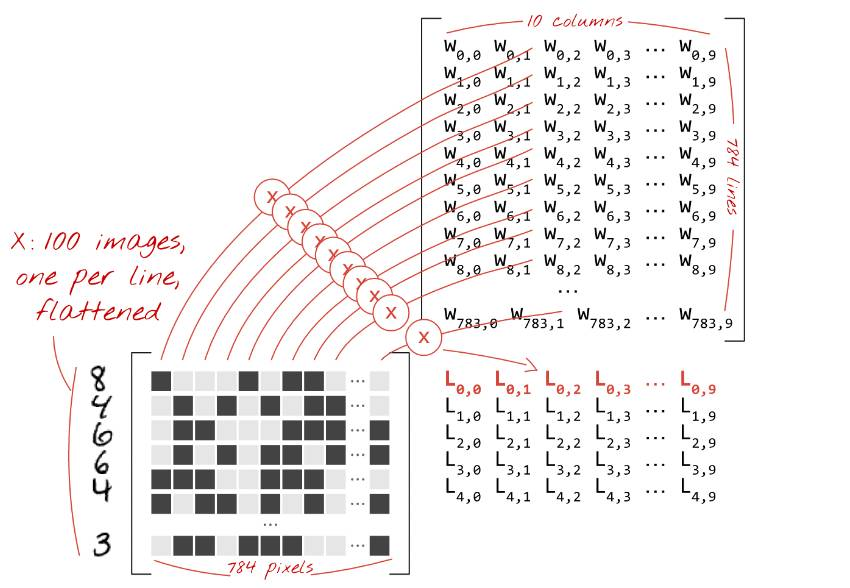「Broadcasting」是 Python 和 numpy（Python 的科学计算库）的一个标准技巧。它扩展了对不兼容维度的矩阵进行正常操作的方式。「Broadcasting add」意味着「如果你因为两个矩阵维度不同的原因而不能将其相加，那么你可以根据需要尝试复制一个小的矩阵使其工作。」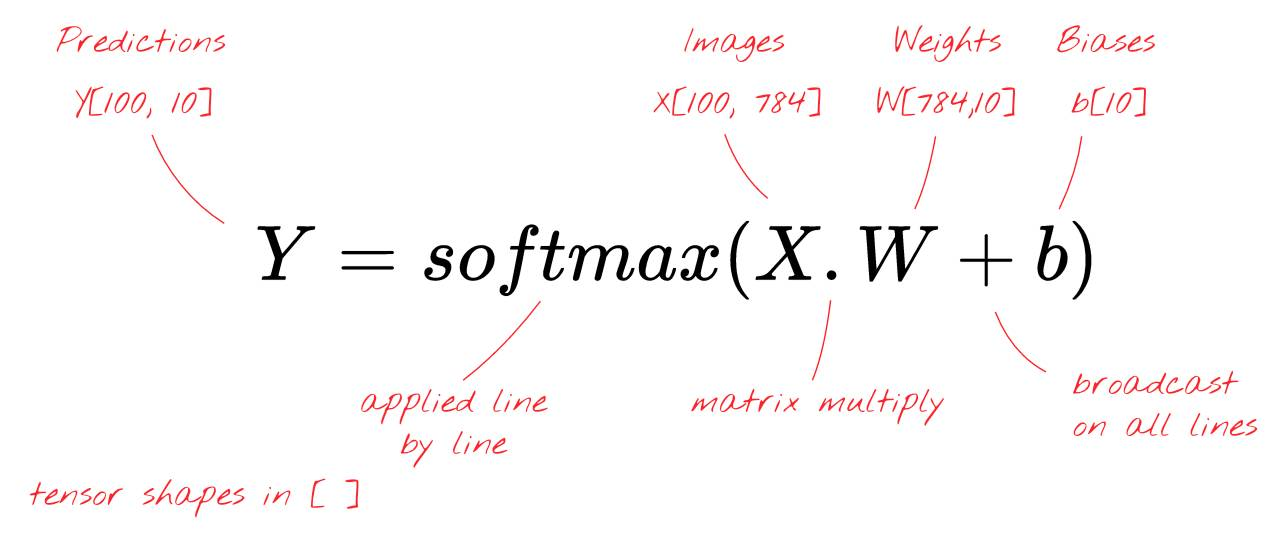「张量（tensor）」像一个矩阵，但是却有着任意数量的维度。一个 1 维的张量是一个向量。一个二维的张量是一个矩阵。然后你可以有 3, 4, 5 或者更多维的张量。

5、理论：梯度下降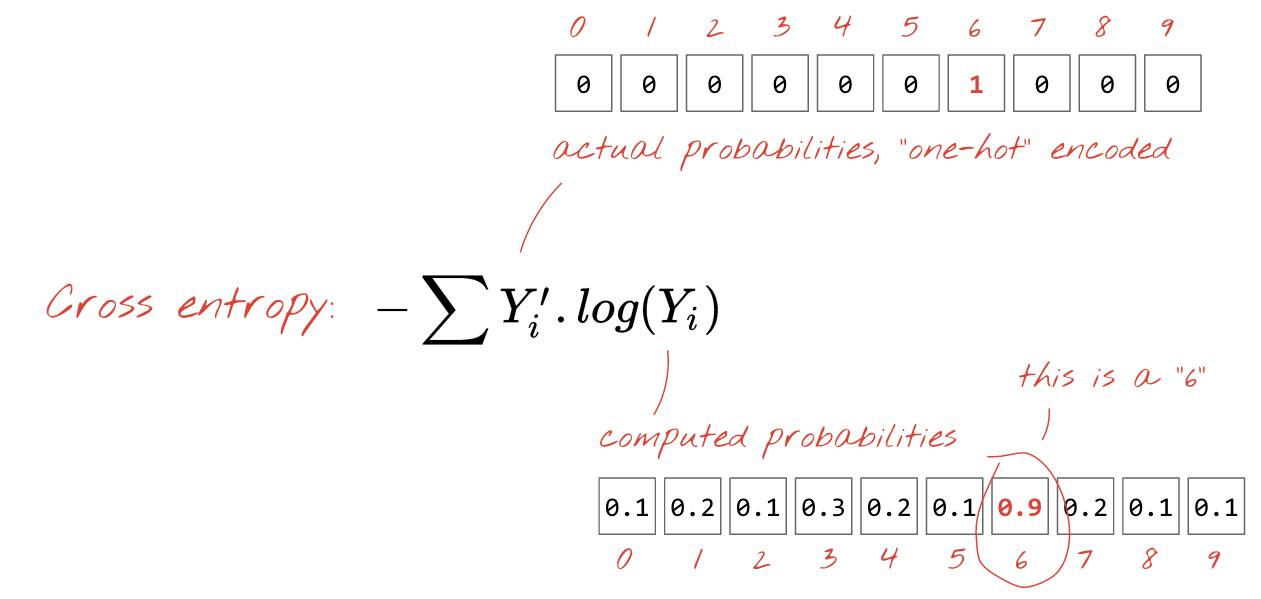「one-hot」编码意味着你使用一个 10 个值的向量，其中除了第 6 个值为 1 以外的所有值都是 0。这非常方便，因为这样的格式和我们神经网络预测输出的格式非常相似，同时它也作为一个 10 值的向量。

「训练」一个神经网络实际上意味着使用训练图像和标签来调整权重和偏置，以便最小化交叉熵损失函数。它是这样工作的。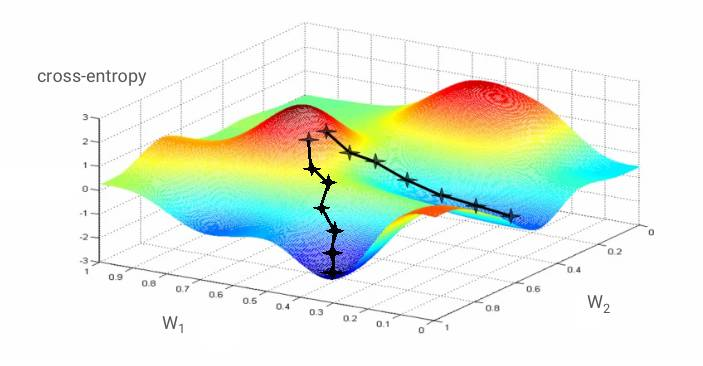「学习率（learning rate）」： 在整个梯度的长度上，你不能在每一次迭代的时候都对权重和偏置进行更新。这就会像是你穿着七里靴却试图到达一个山谷的底部。你会直接从山谷的一边到达另一边。为了到达底部，你需要一些更小的步伐，即只使用梯度的一部分，通常在 1/1000 区域中。我们称这个部分为「学习率（Learning rate）」。

Training digits and labels => loss function => gradient (partial derivatives) => steepest descent => update weights and biases => repeat with next mini-batch of training images and labels

6、实验：让我们来看看代码

mnist_1.0_softmax.py：

https://github.com/martin-gorner/tensorflow-mnist-tutorial/blob/master/mnist_1.0_softmax.py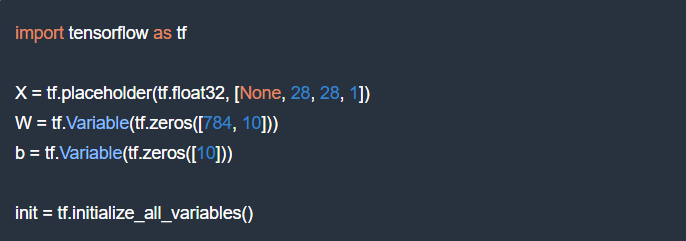• 28, 28, 1: 图像是 28x28 每像素 x 1（灰度）。最后一个数字对于彩色图像是 3 但在这里并非是必须的。

• None: 这是代表图像在小批量（mini-batch）中的数量。在训练时可以得到。

28, 28, 1: 图像是 28x28 每像素 x 1（灰度）。最后一个数字对于彩色图像是 3 但在这里并非是必须的。

None: 这是代表图像在小批量（mini-batch）中的数量。在训练时可以得到。

mnist_1.0_softmax.py：

https://github.com/martin-gorner/tensorflow-mnist-tutorial/blob/master/mnist_1.0_softmax.py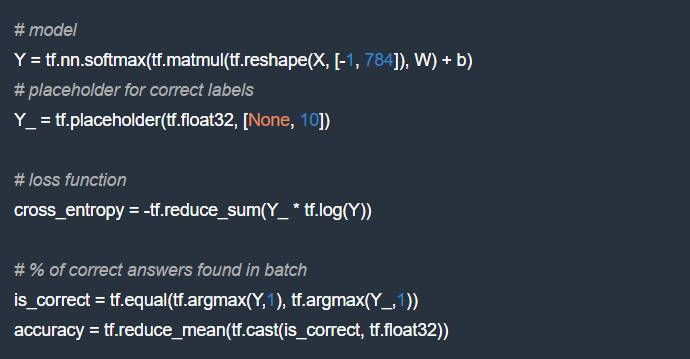mnist_1.0_softmax.py：

https://github.com/martin-gorner/tensorflow-mnist-tutorial/blob/master/mnist_1.0_softmax.py)

optimizer = tf.train.GradientDescentOptimizer(0.003)

train_step = optimizer.minimize(cross_entropy)

TensorFlow 的 “延迟执行（deferred execution）” 模型：TensorFlow 是为分布式计算构建的。它必须知道你要计算的是什么、你的执行图（execution graph），然后才开始发送计算任务到各种计算机。这就是为什么它有一个延迟执行模型，你首先使用 TensorFlow 函数在内存中创造一个计算图，然后启动一个执行 Session 并且使用 Session.run 执行实际计算任务。在此时，图形无法被更改。

mnist_1.0_softmax.py：

https://github.com/martin-gorner/tensorflow-mnist-tutorial/blob/master/mnist_1.0_softmax.py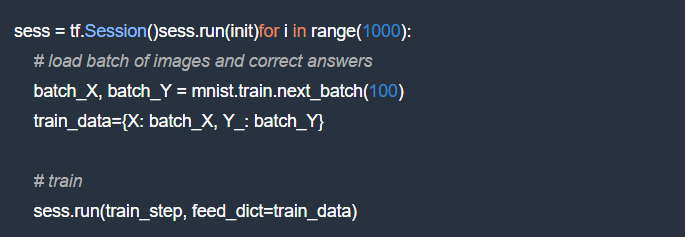# success ?

a,c = sess.run([accuracy, cross_entropy], feed_dict=train_data)

# success on test data ?

test_data={X: mnist.test.images, Y_: mnist.test.labels}

a,c = sess.run([accuracy, cross_entropy], feed=test_data)

TensorFlow 和 Numpy 是朋友：在准备计算图时，你只需要操纵 TensorFlow 张量和命令，比如 tf.matmul, tf.reshape 等。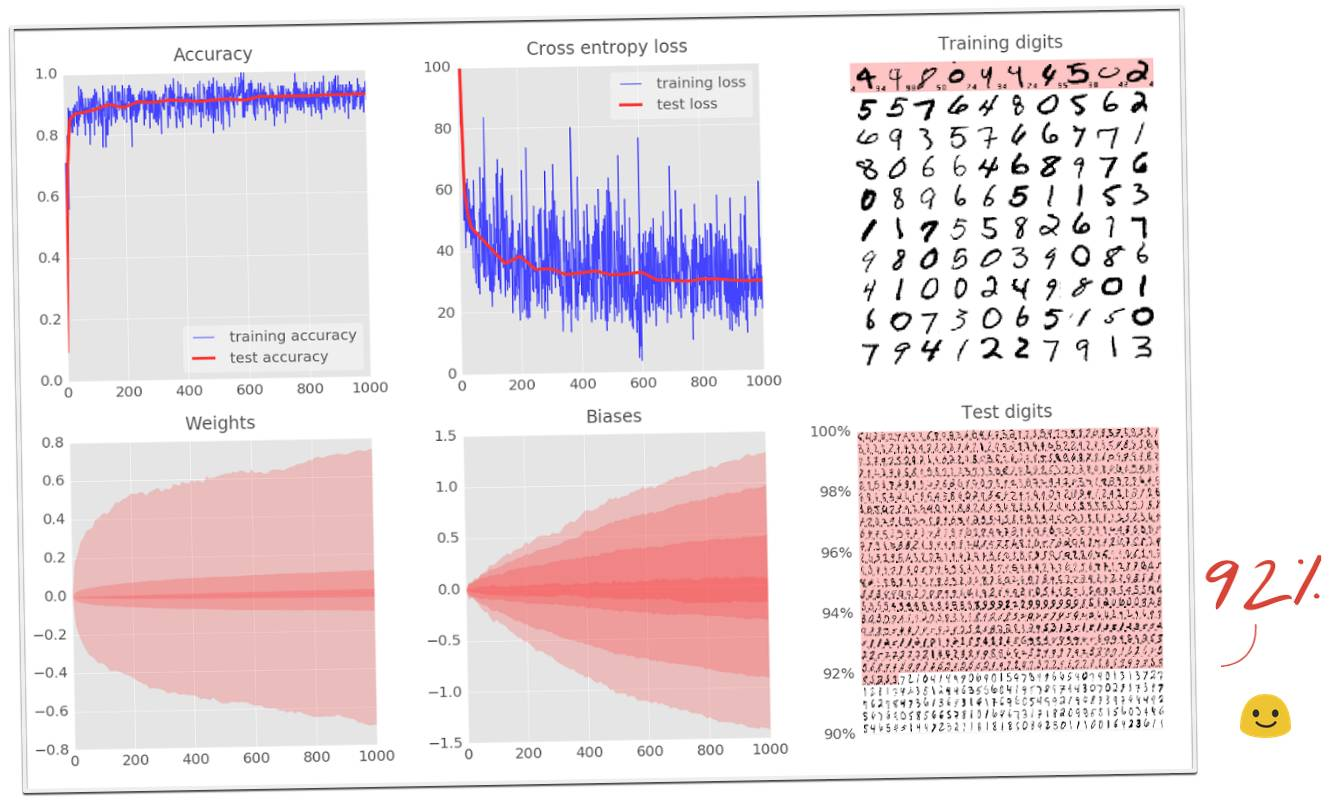7、实验:增加层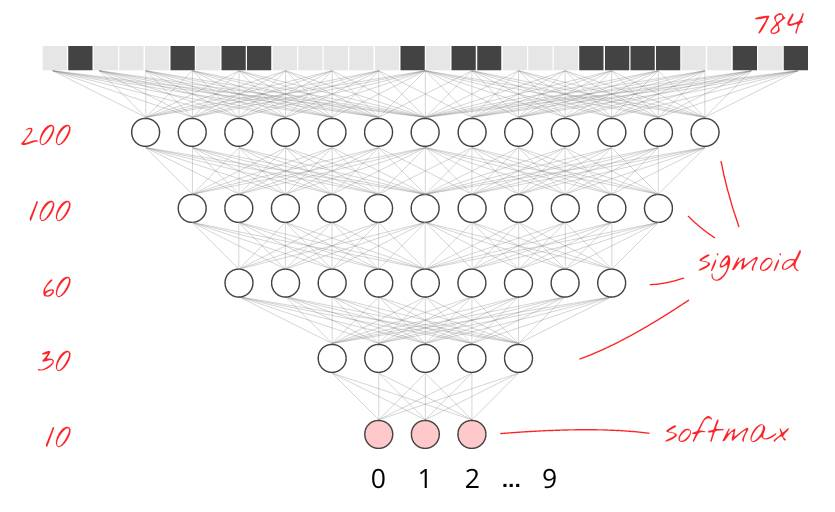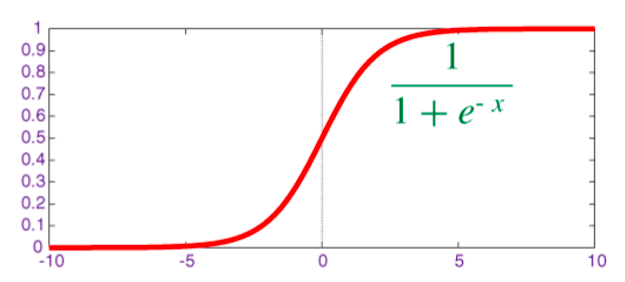W1 = tf.Variable(tf.truncated_normal([28*28, 200] ,stddev=0.1))

B1 = tf.Variable(tf.zeros())

W2 = tf.Variable(tf.truncated_normal([200, 10], stddev=0.1))

B2 = tf.Variable(tf.zeros())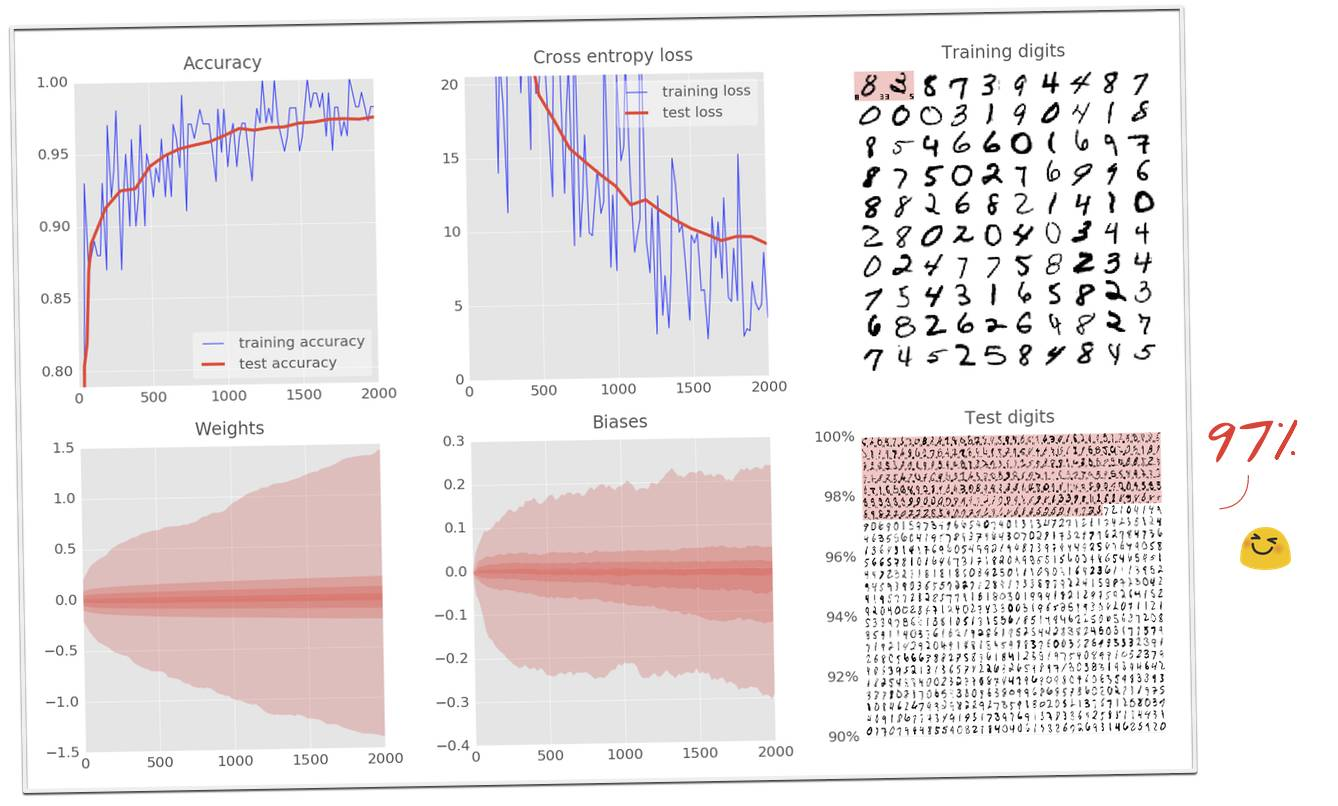8、实验：深度网络需要特别注意的地方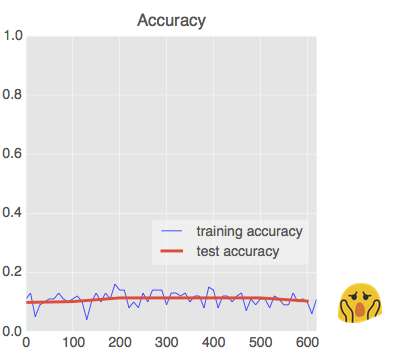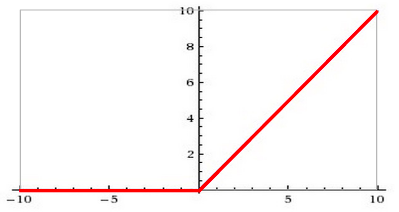W = tf.Variable(tf.truncated_normal([K, L] ,stddev=0.1))

B = tf.Variable(tf.ones([L])/10)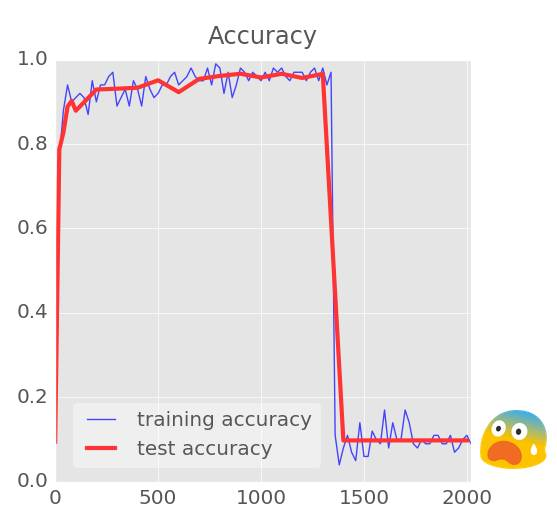Y = tf.nn.softmax(tf.matmul(Y4, W5) + B5)

Ylogits = tf.matmul(Y4, W5) + B5Y = tf.nn.softmax(Ylogits)

cross_entropy = tf.nn.softmax_cross_entropy_with_logits(Ylogits, Y_)

cross_entropy = tf.reduce_mean(cross_entropy)*100

9、实验：学习速率衰退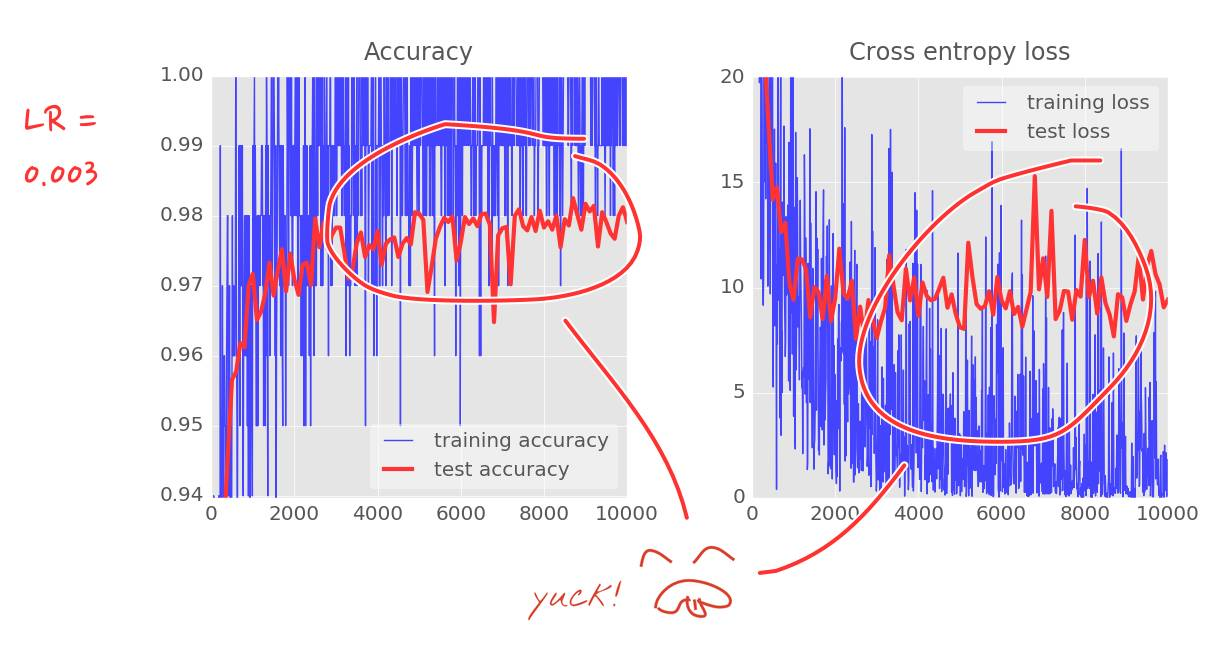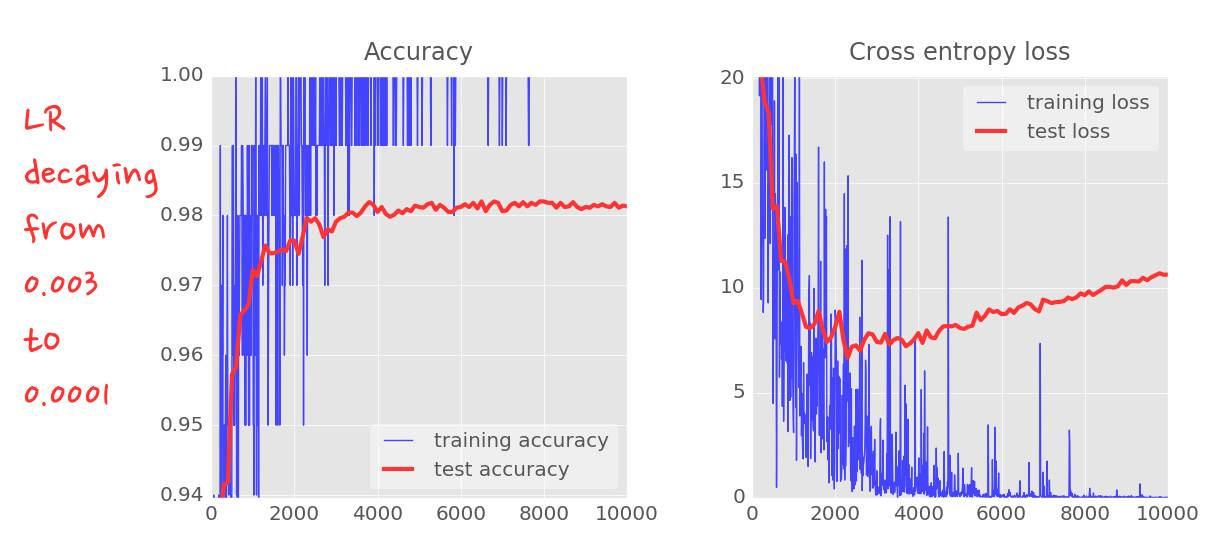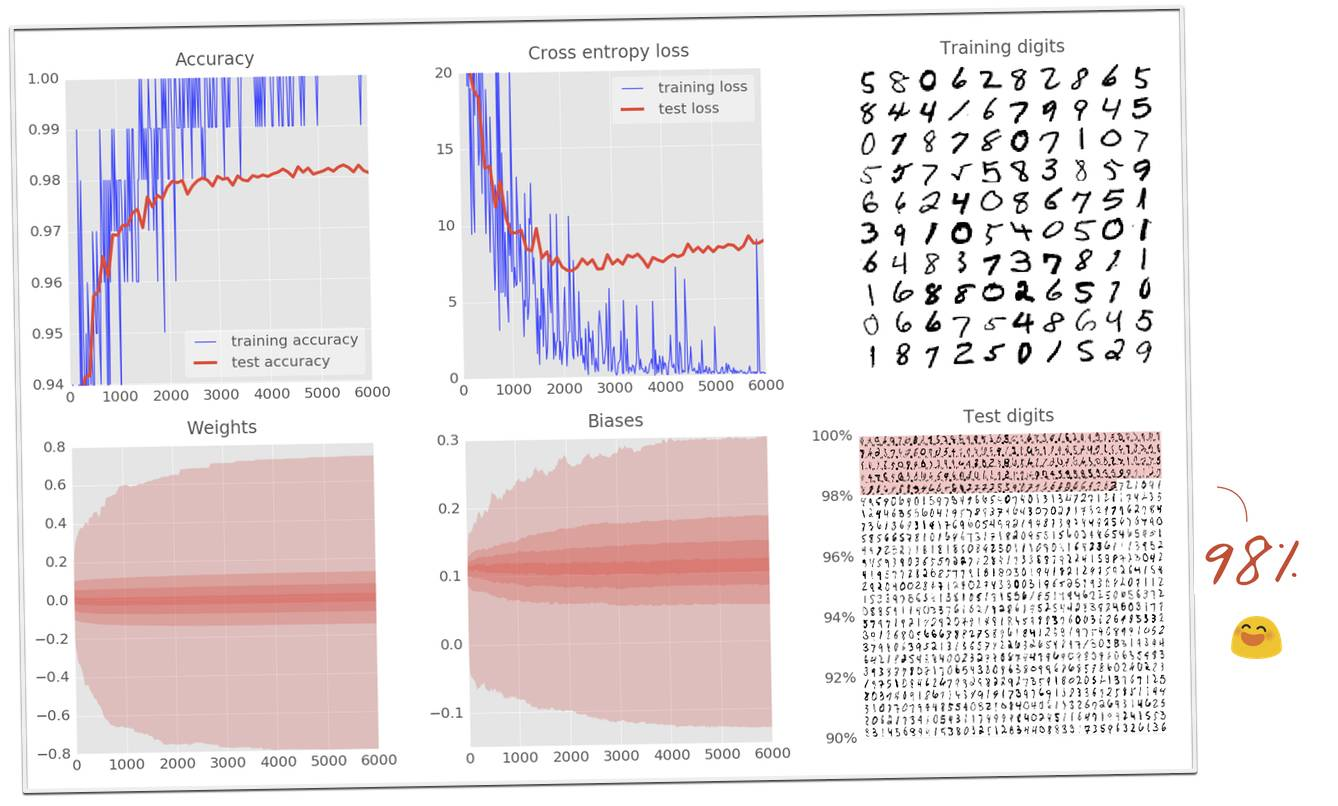10、实验：dropout、过拟合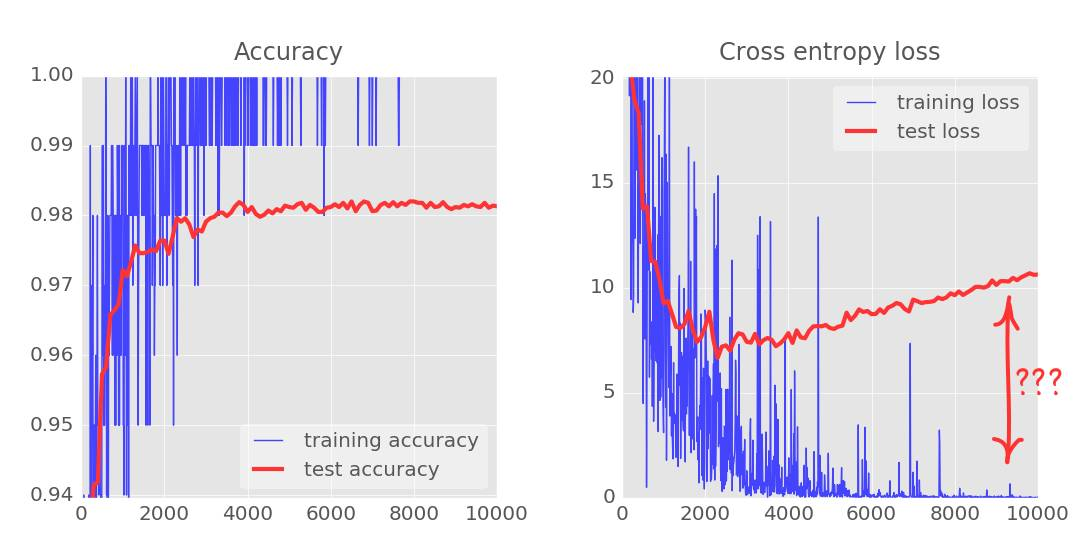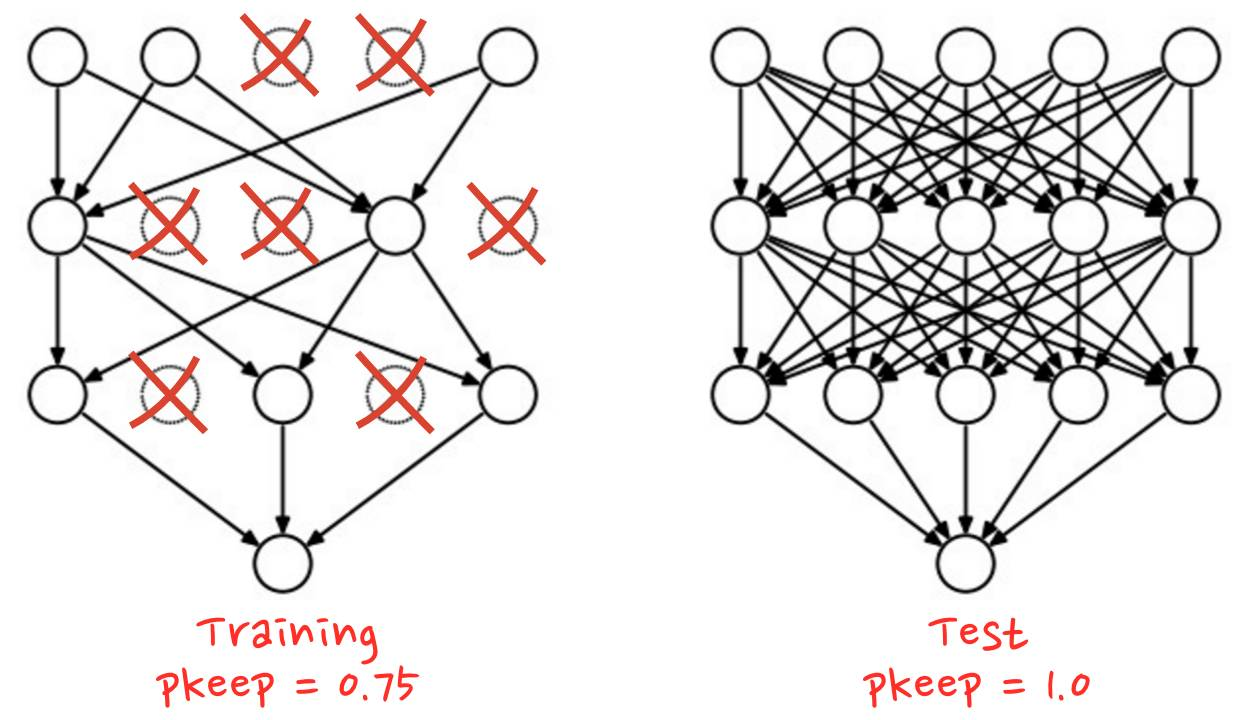TensorFlow 提供一个 dropout 函数可以用在一层神经网络的输出上。它随机地清零一些输出并且把剩下的提升 1/pkeep。这里是如何把它用在一个两层神经网络上的例子。

# feed in 1 when testing, 0.75 when training

pkeep = tf.placeholder(tf.float32)

Y1 = tf.nn.relu(tf.matmul(X, W1) + B1)

Y1d = tf.nn.dropout(Y1, pkeep)

Y = tf.nn.softmax(tf.matmul(Y1d, W2) + B2)

(https://github.com/martin-gorner/tensorflow-mnist-tutorial/blob/master/mnist_2.2_five_layers_relu_lrdecay_dropout.py)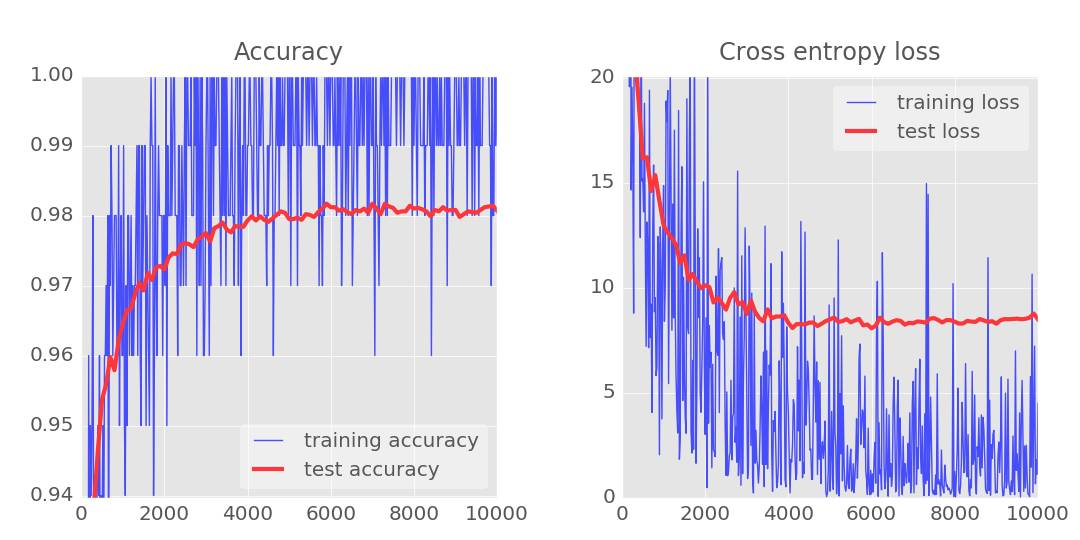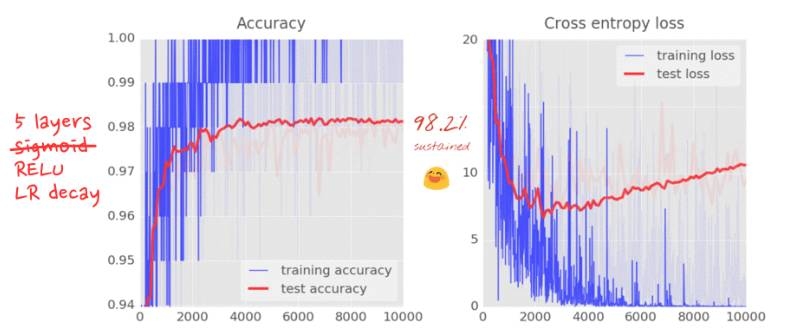11、理论：卷积网络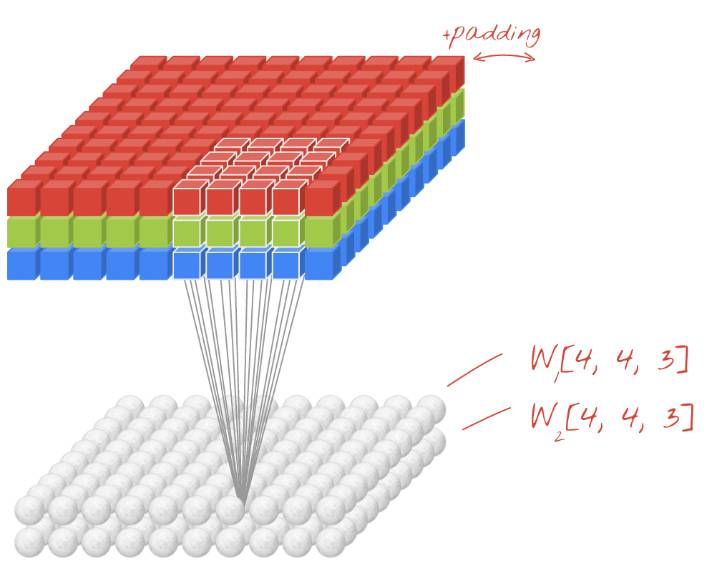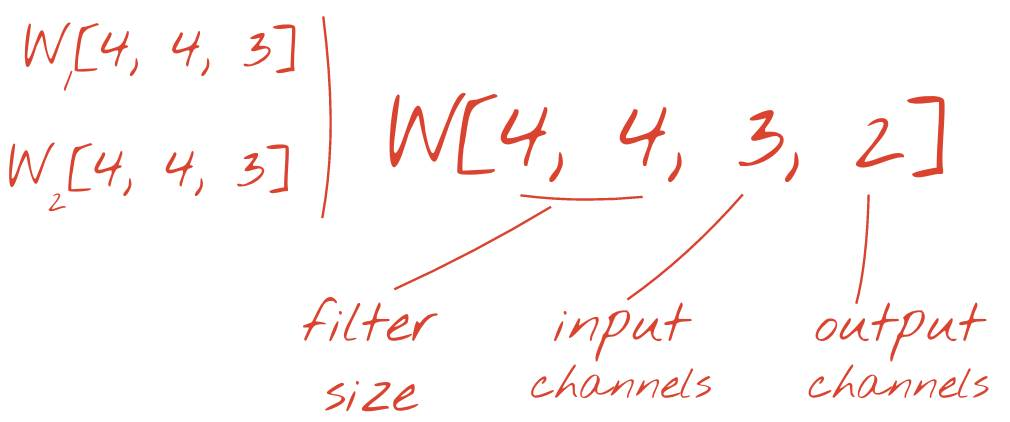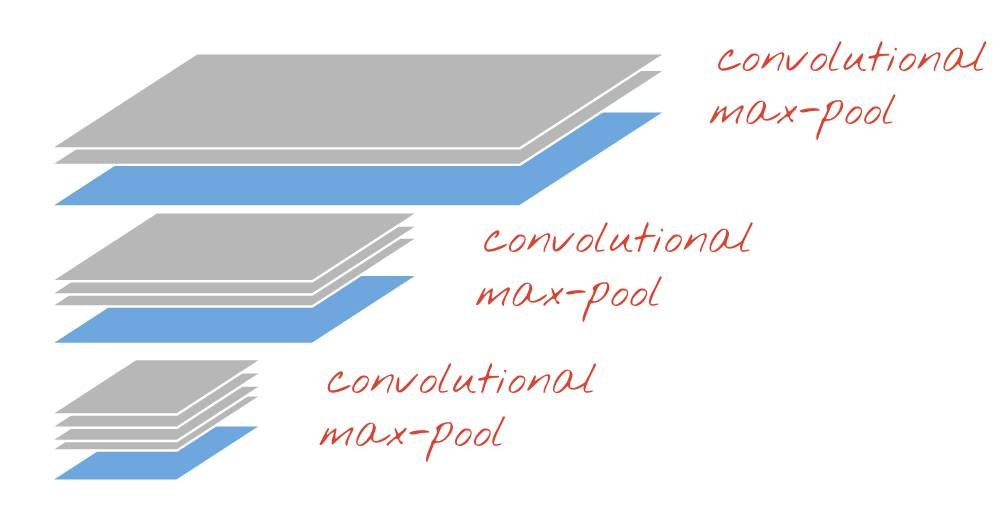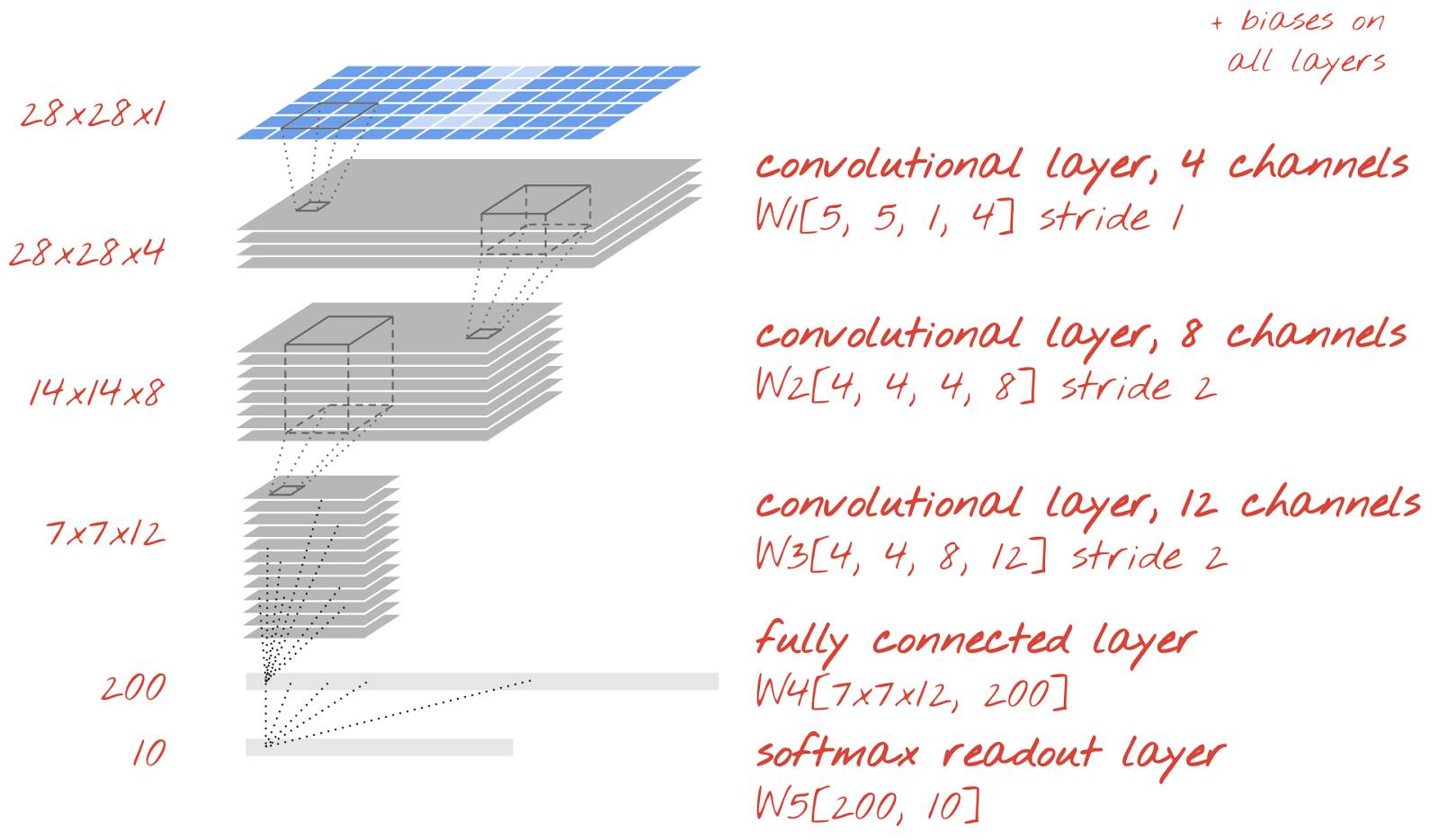12、实现：一个卷积网络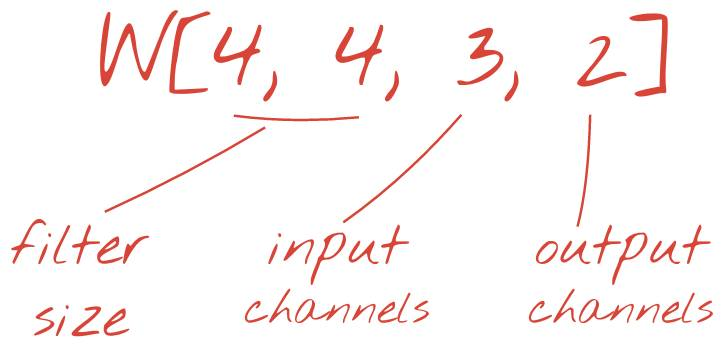W = tf.Variable(tf.truncated_normal([4, 4, 3, 2], stddev=0.1))

B = tf.Variable(tf.ones()/10) # 2 is the number of output channels

stride = 1 # output is still 28x28

Ycnv = tf.nn.conv2d(X, W, strides=[1, stride, stride, 1], padding='SAME')

Y = tf.nn.relu(Ycnv + B)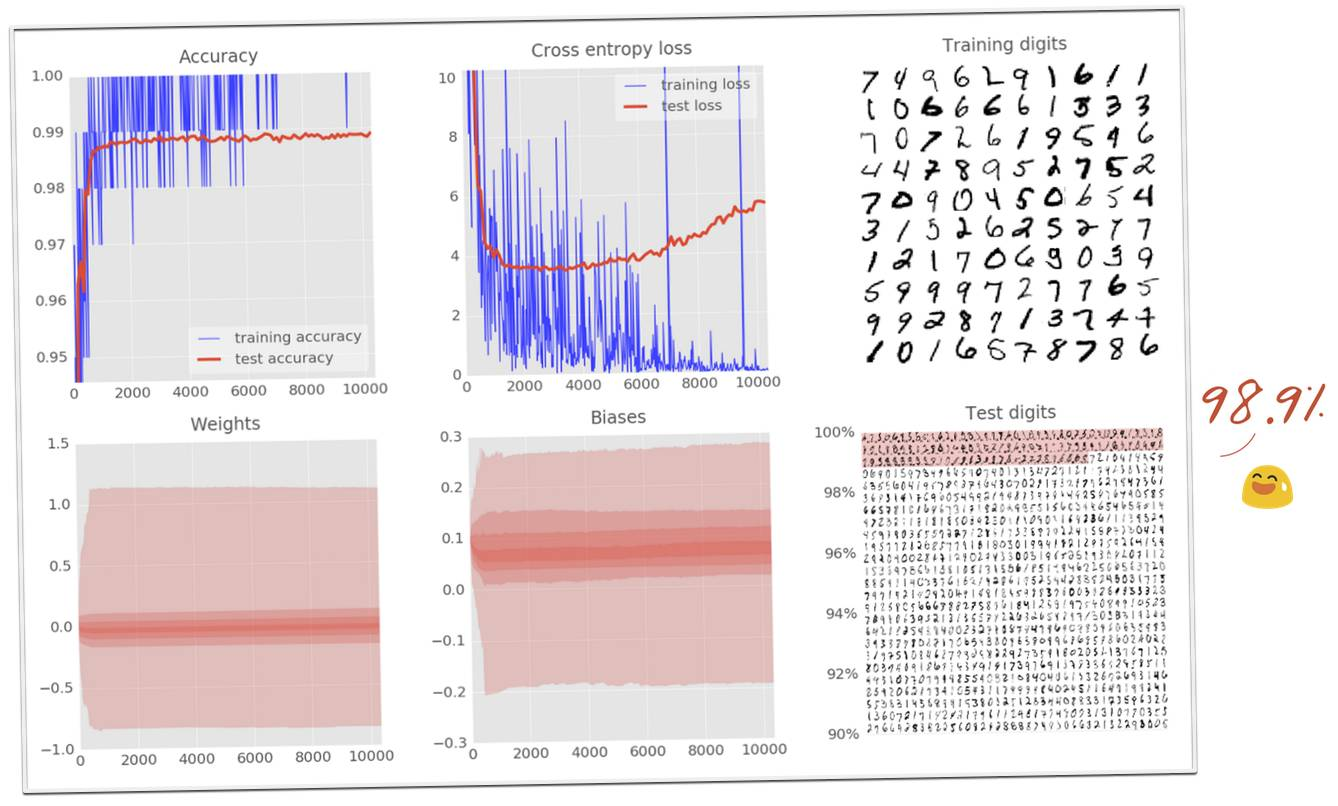13、99% 准确率的挑战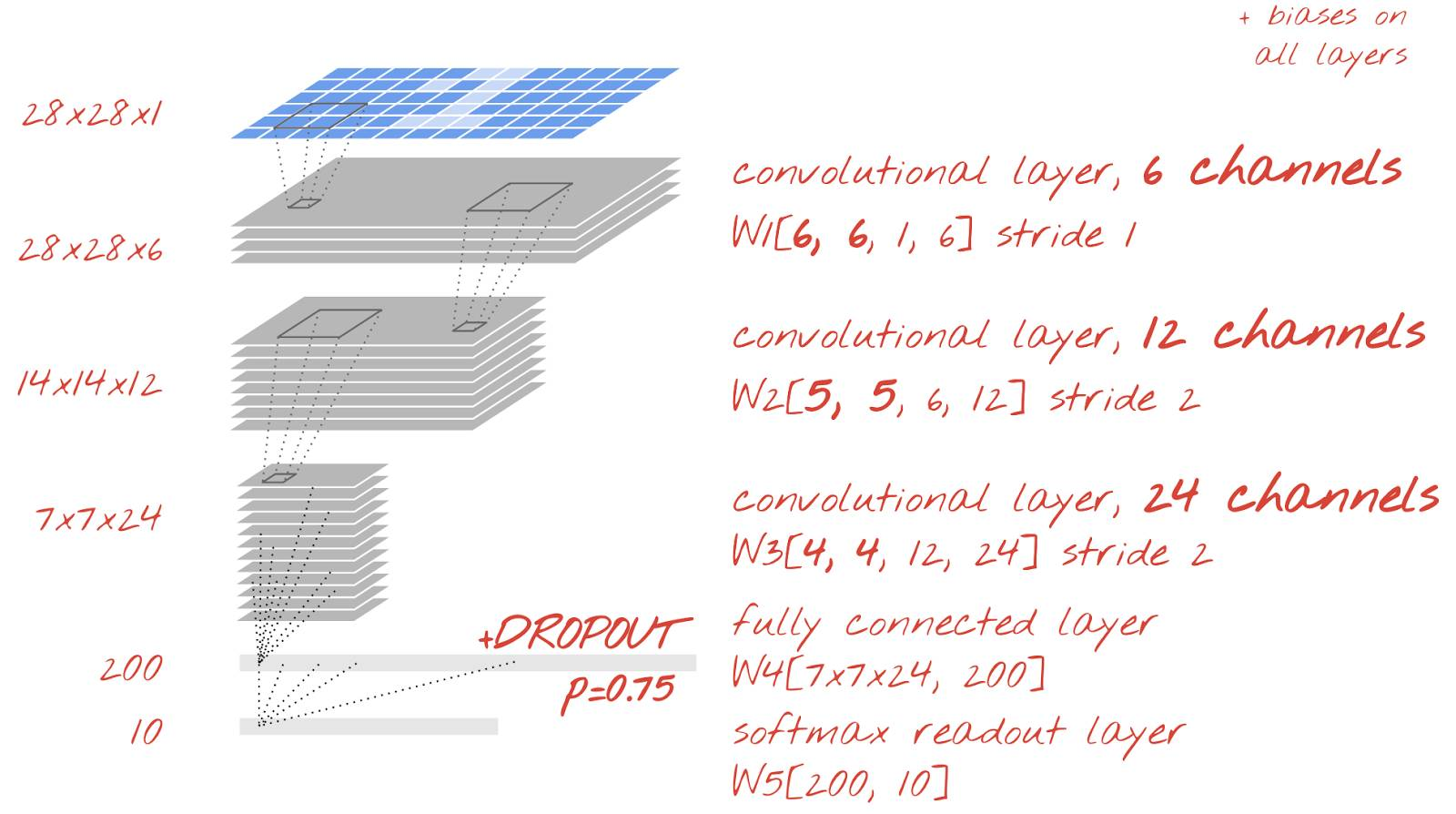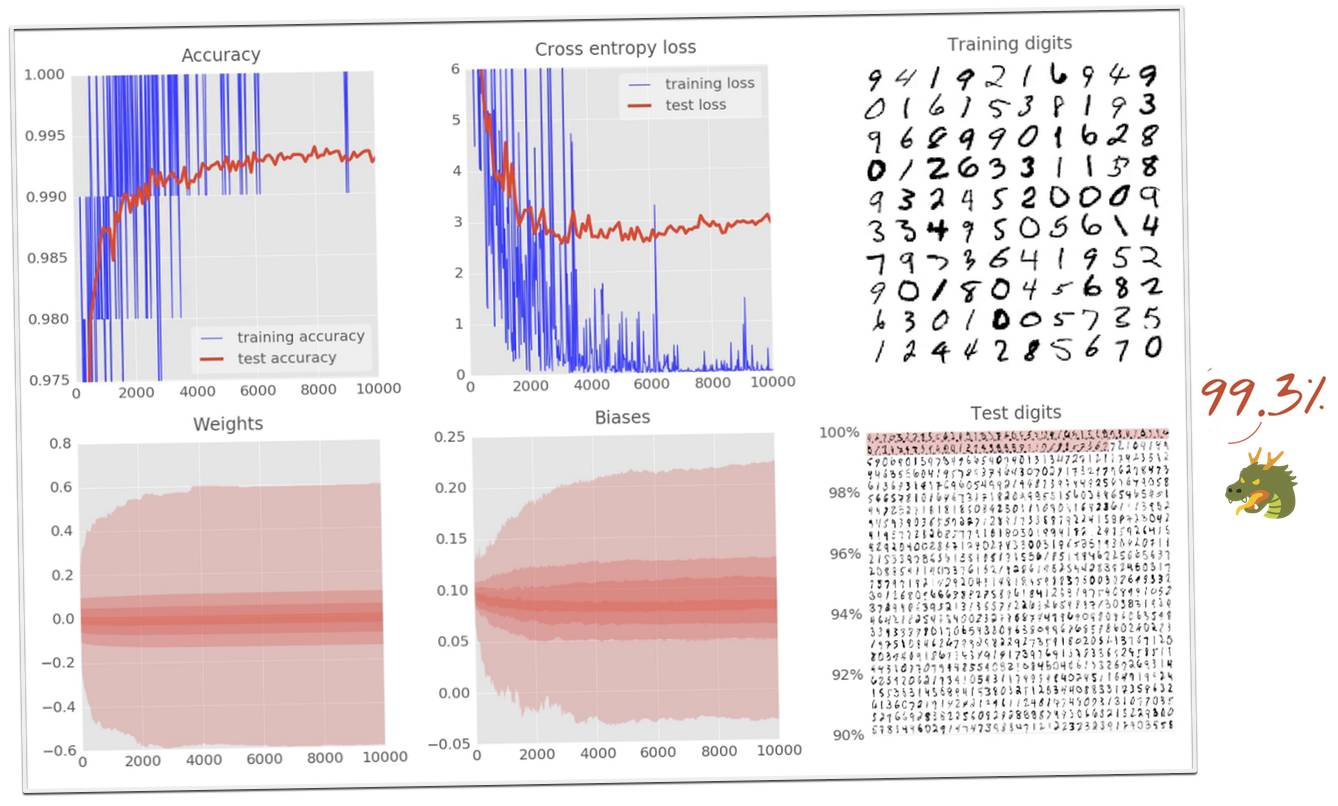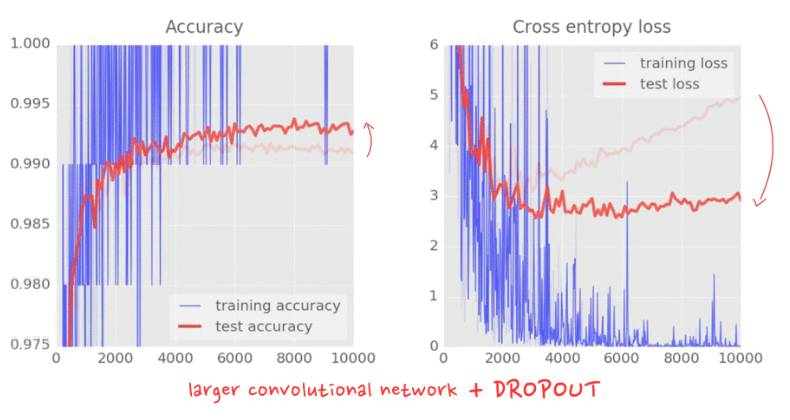14、恭喜！• 在完成了完全神经网络和卷积网络后，你应该学习循环神经网络：https://www.tensorflow.org/tutorials/recurrent/。

• 在本教程中，你已经学习了如何在矩阵层次构建 TensorFlow 模型。Tensorflow 还有更高级的 API，称为 tf.learn：https://www.tensorflow.org/tutorials/tflearn/

• 要在云上的分布式框架上训练，我们提供 Cloud ML 服务：https://cloud.google.com/ml

• 最后，我们希望收到你的反馈。如果你在发现了本实验中的些许错误，或者你认为有什么需要改进的地方，请告诉我们。我们通过 GitHub 处理反馈。反馈链接：https://github.com/googlecodelabs/feedback/issues/new?title=[cloud-tensorflow-mnist]:&labels[]=content-platform&labels[]=cloud

©本文为机器之心编译，转载请联系本公众号获得授权

✄------------------------------------------------